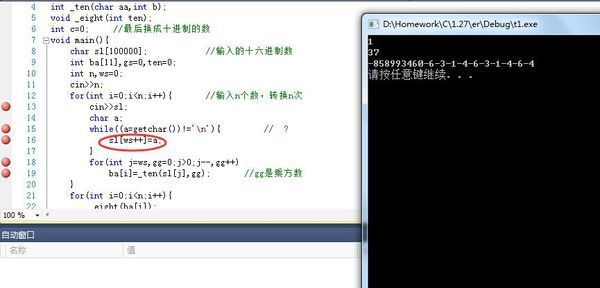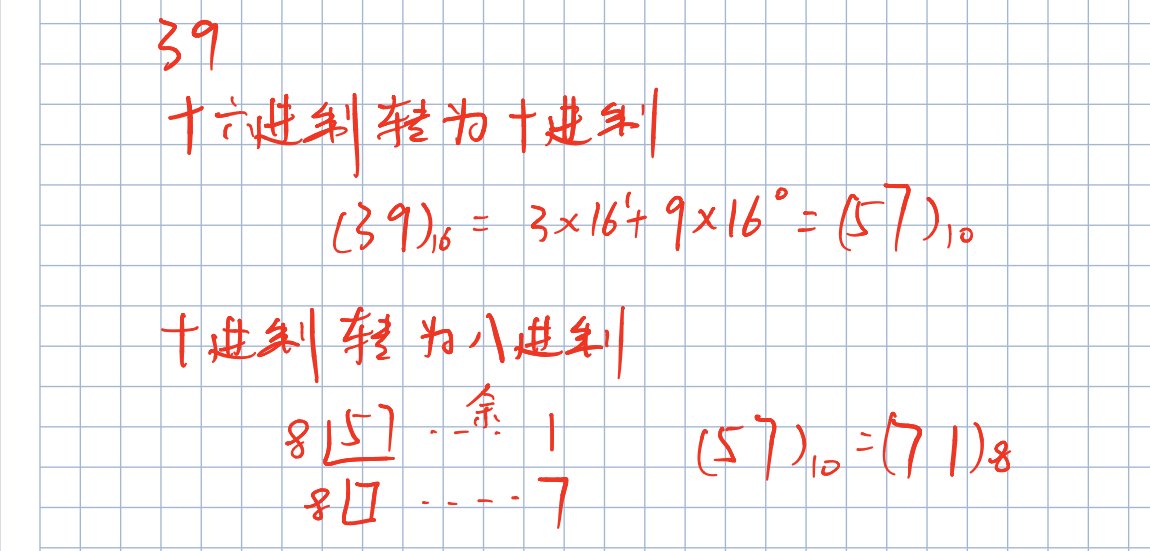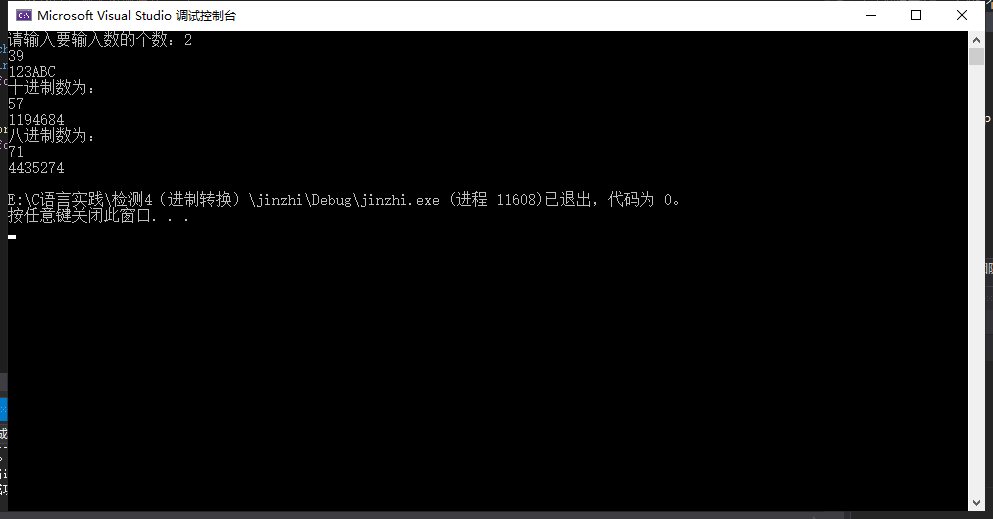• 十六进制转八进制：给定n个十六进制整数输出它们对应的八进制数。
2022-02-05 12:30:22

问题描述
给定n个十六进制正整数，输出它们对应的八进制数。

输入格式
输入的第一行为一个正整数n （1<=n<=10）。
接下来n行，每行一个由0~9、大写字母A~F组成的字符串，表示要转换的十六进制正整数，每个十六进制数长度不超过100000。

输出格式
输出n行，每行为输入对应的八进制正整数。

【注意
输入的十六进制数不会有前导0，比如012A。
输出的八进制数也不能有前导0。

样例输入
2
39
123ABC

样例输出
71
4435274

package com.test01.xyx;

import java.util.Scanner;

/**
* @author yuxing
* @create 2022-02-05-12:18
* @content:给定n个十六进制正整数，输出它们对应的八进制数。
*/
public class Test07 {

public static void main(String[] args) {
Scanner sc = new Scanner(System.in);
//获得输入16进制数的个数
int count = sc.nextInt();

if (count >= 1 && count <= 10) {
//创建一个数组
int[] arr = new int[count];
//对每一个数进行操作
for (int i = 0; i < count; i++) {
//获得输入的16进制数字符串
String strnum = sc.next();
//转换为16进制
Integer hexnum = Integer.valueOf(strnum, 16);
System.out.println(hexnum);
//转换为8进制字符串
String num = Integer.toOctalString(hexnum);
//转换为Int类型
int result = Integer.parseInt(num);
//存入数组
arr[i] = result;
}
//遍历数组输出
for (int i = 0; i < arr.length; i++) {
System.out.println(arr[i]);
}
}
}
}java 算法
更多相关内容
• 　给定n个十六进制整数输出它们对应的八进制数。 输入格式 　输入的第一行为一个正整数n （1<=n<=10）。 　接下来n行，每行一个由09、大写字母AF组成的字符串，表示要转换的十六进制整数，每个十六进制...
• 本文实例讲述了C++实现十六进制字符串转换为十进制整数的方法。分享给大家供大家参考。具体实现方法如下： /* * 将十六进制数字组成的字符串（包含可选的前缀0x或0X）转换为与之等价的整型值 */ #include #include...
•c++
• 问题描述给定n个十六进制整数输出它们对应的八进制数。输入格式输入的第一行为一个正整数n (1<=n<=10)。接下来n行，每行一个由0~9、大写字母A~F组成的字符串，表示要转换的十六进制整数，每个十六进制数...

问题描述

给定n个十六进制正整数，输出它们对应的八进制数。

输入格式

输入的第一行为一个正整数n (1<=n<=10)。

接下来n行，每行一个由0~9、大写字母A~F组成的字符串，表示要转换的十六进制正整数，每个十六进制数长度不超过100000。

输出格式

输出n行，每行为输入对应的八进制正整数。

注意

输入的十六进制数不会有前导0，比如012A。

输出的八进制数也不能有前导0。

下面是我的程序：

#include

#include

using namespace std;

int _ten(char aa,int b);

void _eight(int ten);

int c=0;     //最后换成十进制的数

void main(){

char sl;//输入的十六进制数

int ba,gs=0,ten=0;

int n,ws=0;

cin>>n;

for(int i=0;i

cin>>sl;

char a;

while((a=getchar())!='\n'){//  ?

sl[ws++]=a;

}

for(int j=ws,gg=0;j>0;j--,gg++)

ba[i]=_ten(sl[j],gg);//gg是乘方数

}

for(int i=0;i

_eight(ba[i]);

}

system("pause");

}

int _ten(char aa,int b){

int a;

if(aa=='a'||aa=='A')

a=10;

else if(aa=='b'||aa=='B')

a=11;

else if(aa=='c'||aa=='C')

a=12;

else if(aa=='d'||aa=='D')

a=13;

else if(aa=='e'||aa=='E')

a=14;

else if(aa=='F'||aa=='f')

a=15;

else a=aa-'0';

int d=1;

if(b==0)

c+=a;

else{

for(int i=0;i

d*=16;

c+=a*d;

}

return c;

}

void _eight(int ten){

int a,i=0;

for(i=0;;i++){

if(ten==0)break;

a[i]=ten%8;

ten=ten/8;

}

for(int j=i;j>=0;j--)

cout<

cout<

}直接没用运行while语句里的那一句。getchar()到底怎么用呢，  求高手赐教~

这个程序是对的吗？0.0

展开全文• 给定n个十六进制整数输出它们对应的八进制数。 要求 输入格式：输入的第一行为一个正整数n （1<=n<=10）。 　 接下来n行，每行一个由0-9 　 、大写字母A~F组成的字符串，表示要 转换的十六进制正...

# 问题描述

给定n个十六进制正整数，输出它们对应的八进制数。


要求

输入格式：输入的第一行为一个正整数n （1<=n<=10）。
接下来n行，每行一个由0-9
、大写字母A~F组成的字符串，表示要 转换的十六进制正整数，每个十六进制数长度不超过100000。
输出格式：输出n行，每行为输入对应的八进制正整数。
分析
要将十六进制转换成八进制需要先将十六进制转换成十进制，再将十进制转换为八进制。转换过程如下图：由十进制转换八进制可以看出先得到的数为低位，所以这里将八进制的数先推入栈中，待得到结果后再依次弹出。

#define _CRT_SECURE_NO_WARNINGS  //不定义这个scanf总报错
#include<stdio.h>
#include<stdlib.h>
#include<string.h>
#include<math.h>

#define MAXSIZE 1024
#define INFINITY 65535
//定义一个栈存放八进制数
typedef struct SeqStack_1{
int data[MAXSIZE];
int top;
}SeqStack;
int IsEmpty(SeqStack* stack) { //判断栈是否为空
if (stack->top == -1)
return 1;
else
return 0;
}
void InitStack(SeqStack* stack) {  //栈的初始化
stack->top = -1;
}
void SeqStack_Push(SeqStack* stack, int val) {  //将数据压入栈中
if (stack->top >= MAXSIZE - 1)
return;
stack->top++;
stack->data[stack->top] = val;
}
int SeqStack_Pop(SeqStack* stack) { //弹出栈
if(!IsEmpty(stack))
return stack->data[stack->top--];
return INFINITY;//返回无穷大
}
int main() {
int n,len, sum = 0, num, ten;
printf("请输入要输入数的个数：");
scanf_s("%d ", &n);
if (n > 10) {
printf("输入参数有误！");
return 0;
}
char a;//定义一个二位数组存放字符串
int b;
for (int i = 0; i < n; i++) {  //确定要转换的个数
scanf("%s", a[i]);
}
printf("十进制数为：\n");
for (int i = 0; i < n; i++) {  //取某一个字符串
len = strlen(a[i]);
for (int j = 0; j < len; j++) {  //读取一个字符串中的具体值
switch (a[i][j]) {
case'A':num = 10; break;
case'B':num = 11; break;
case'C':num = 12; break;
case'D':num = 13; break;
case'E':num = 14; break;
case'F':num = 15; break;
default:num = (int)a[i][j]-48; break;//直接强制类型转出的时ASCII码，故减去48
}
sum = sum + num * (int)pow(16, len - j - 1);//十六进制转化为十进制
}
b[i] = sum;
sum = 0;

printf("%d \n", b[i]);
}
printf("八进制数为：\n");
//将十进制转换为八进制
SeqStack stack;  //定义栈
InitStack(&stack);//初始化栈
for (int i = 0; i < n; i++) {
ten = b[i];
while (ten != 0) {     //十进制转换至八进制，并将数据压入栈
SeqStack_Push(&stack, ten % 8);
ten = ten / 8;
}
while ((&stack)->top >= 0) {
printf("%d", SeqStack_Pop(&stack));//依次弹出栈
}
InitStack(&stack);
printf("\n");
}
return 0;
}


运行结果如下：展开全文c语言
• 问题描述　给定n个十六进制整数输出它们对应的八进制数。 输入格式 　输入的第一行为一个正整数n （1<=n<=10）。 　接下来n行，每行一个由09、大写字母AF组成的字符串，表示要转换的十六进制整数，每个...

1.题目
问题描述　给定n个十六进制正整数，输出它们对应的八进制数。
输入格式
输入的第一行为一个正整数n （1<=n<=10）。
接下来n行，每行一个由09、大写字母AF组成的字符串，表示要转换的十六进制正整数，每个十六进制数长度不超过100000。
输出格式: 输出n行，每行为输入对应的八进制正整数。
【注意】 输入的十六进制数不会有前导0，比如012A。 输出的八进制数也不能有前导0。
样例输入
2
39
123ABC
样例输出
71
4435274
【提示】
先将十六进制数转换成某进制数，再由某进制数转换成八进制。
2.思路

1. 自己的思路：看了题目提示“先将十六进制数转换成某进制数，再由某进制数转换成八进制。”想的就是先将十六进制转成十进制，再将十进制转成八进制，自己写了一遍。但是提交过后却是错误零分。
内联代码片
public class HexToOctal {

public static void main(String[] args) {
Scanner sc = new Scanner(System.in);
int m = sc.nextInt();
ArrayList<String> s = new ArrayList<String>();

for(int i = 0; i < 2 * m - 1; i++) {
String a = sc.nextLine();
if(a.length() == 0) {
continue;
}else
}
convertToDeciamalAndOctal(s);
}
public static void convertToDeciamalAndOctal(ArrayList<String> s) {
int[] arr = new int[s.size()];

for(int i = 0; i < s.size(); ++i) {
arr[i] = Integer.parseInt(s.get(i), 16);
}

for (int i = 0; i < arr.length; i++) {
System.out.println(Integer.toOctalString(arr[i]));
}

}
}

1. 后面参考了其他博主的文章，意识到了自己的错误是“每个十六进制数长度不超过100000。”稍微大一点的十六进制连long都无法表示了。此时思路就是将十六进制先转成二进制，再用二进制转成八进制，并且都以字符串存储，这样避免无法存储的情况。
难点体会及注意事项：
（1）注意从键盘获取数据时， sc.nextLine(); 和 sc.next() 的区别，最好是采用后者，因为我发现用 sc.nextLine()时会将换行符也读入，导致后面还需单独处理，而 sc.next()就能屏蔽换行符之类的带来的影响。
（2）不断追加字符串时最好使用StringBuffer或者StringBuilder，但是这样用String接收返回的StringBuffer或者StringBuilder时候就要注意调用toString方法，当时没自己写的时候看博主的，还认为toString可有可无。
（3）StringBuffer线程不安全但速度快，StringBuilder线程安全相对StringBuilder就会慢一点点
（4） 内联代码片
import java.util.Scanner;

public class HexToOctal_2 {
//创建全局变量
static String[] bin = {"0000","0001","0010","0011","0100","0101","0110","0111",
"1000","1001","1010","1011","1100","1101","1110","1111"};
static String[] oct = {"0","1","2","3","4","5","6","7"};

public static void main(String[] args) {
Scanner sc = new Scanner(System.in);
int m = sc.nextInt();
String[] a = new String[m];
for(int i = 0; i < m; ++i) {
a[i] = sc.next();
}
for(int i = 0; i < m; ++i) {
/*
* hexToBin(a[i]).toString()   十六进制转二进制
* binToOct(hexToBin(a[i]).toString()).toString() 二进制转八进制
*/
String res1 = binToOct(hexToBin(a[i]).toString()).toString();
if(res1.startsWith("0") ) {
System.out.println(res1.substring(1));
} else
System.out.println(res1);
}

}

//十六进制转二进制（取出数值）
public static StringBuilder hexToBin(String s) {
StringBuilder sb = new StringBuilder();
int start = 0;
int end = 1;//一位16进制数转化成思位二进制数,即 1,2,4,8
while(end <= s.length()) {
String str = transformToBin(s.substring(start, end));
start += 1;
end += 1;
sb.append(str);
}
return sb;
}
//十六进制转二进制（将取出的数字转化成二进制）
public static String transformToBin(String s) {
String b = null;
switch(s) {
case "0": b = bin; break;
case "1": b = bin; break;
case "2": b = bin; break;
case "3": b = bin; break;
case "4": b = bin; break;
case "5": b = bin; break;
case "6": b = bin; break;
case "7": b = bin; break;
case "8": b = bin; break;
case "9": b = bin; break;
case "A": b = bin; break;
case "B": b = bin; break;
case "C": b = bin; break;
case "D": b = bin; break;
case "E": b = bin; break;
case "F": b = bin; break;
}
return b;
}

//二进制转八进制（取出三个二进制）1 2 4 = 7
public static StringBuilder binToOct(String s) {
StringBuilder sb = new StringBuilder();
int start = 0;
int end = 3;
if(s.length() % 3 == 1) {
s = "00" + s;
}
if(s.length() % 3 == 2) {
s = "0" + s;
}
while(end <= s.length()) {
String str = transformToOct(s.substring(start, end));
start += 3;
end += 3;
sb.append(str);
}
return sb;
}
public static String transformToOct(String s) {
String b = new String();
switch(s) {
case "000":  b = oct;  break;
case "001":  b = oct;  break;
case "010":  b = oct;  break;
case "011":  b = oct;  break;
case "100":  b = oct;  break;
case "101":  b = oct;  break;
case "110":  b = oct;  break;
case "111":  b = oct;  break;
}
return b;
}

}


1. 上面的方法写出来之后感觉还是有点复杂，一百来行代码呢，然后我就继续找啊找，主要也不太看得懂c++的方法，找呀找还真逛到了更简单的方法，然后迫不及待的提交去测试，啪，满分通过，神了。API真神奇居然还有BigInteger这东西。

下面展示一些 内联代码片

import java.math.BigInteger;
import java.util.Scanner;

public class HexToOctal_3 {

public static void main(String[] args) {
Scanner sc = new Scanner(System.in);
int m = sc.nextInt();
String[] a = new String[m];
for(int i = 0; i < m; ++i) {
a[i] = sc.next();
}
for(int i = 0; i < m; ++i) {
System.out.println(new BigInteger(a[i],16).toString(8));
}

}
}



我的理解：

1. BigInteger(a[i],16)，16是告诉编译器a[i]是一个十六进制的String类型的数，然后让它转化成十进制。
API：2. toString(8))，将十进制数转化成八进制数。
API：只能感叹API的伟大了。。。。
参考文章：

学习心得：神了。又是秃头的一天

展开全文java 字符串
• 给定n个十六进制整数输出它们对应的八进制数。 输入的十六进制数不会有前导0，比如012A。 输出的八进制数也不能有前导0。 样例输入 　2 　39 　123ABC 样例输出 　71 　4435274 import java.util.Scanner;...java
• 　给定n个十六进制整数输出它们对应的八进制数。输入格式 　输入的第一行为一个正整数n （1<=n<=10）。 　接下来n行，每行一个由0~9、大写字母A~F组成的字符串，表示要转换的十六进制整数，每个十六进制...
• 满意答案hufei11132013.08.21采纳率：53%等级：11已帮助：8071人#include main(){char b={"0123456789ABCDEF"};int c,d,i=0,base=16;long n;printf("Enter a number:\n...do //这个循环是要把十进制分离成十...
• 　给定n个十六进制整数输出它们对应的八进制数。 输入格式 　输入的第一行为一个正整数n （1<=n<=10）。 　接下来n行，每行一个由09、大写字母AF组成的字符串，表示要转换的十六进制整数，每个十六进制...c++
• printf("请输入一个任意的四位十六进制整数：\n"); scanf("%4x",&num); a = num >> 12; //最高位变成最低位 b = (num & 0x0f00) >> 4; //第三位置换成第二位（从左往右） c = ...
• //因为八进制和十六进制换成二进制的最小公倍数位12.及3个16进制数 if ( l % 3 == 1 ) { add = "00" ; str = add + str ; } else if ( l % 3 == 2 ) { add = "0" ; str = add + str ; } ...编程语言 c++
• 以十六进制输出 代码示例 #include<iostream> using namespace std; int main(){ int a; scanf("%d",&a); printf("原始数据：%d \n",a); printf("八进制输出：%o \n",a); printf("以十六...c++ c语言
• 读取一个整数二进制、八进制和十六进制输出十六进制浮点数输出倒数。 */ package com.javaexercixe.java; import java.util.Scanner; public class FirstExercise { public static void main(String[] ...
• 一:目的将十进制整数转化为二进制与十六进制输出。二:技术。1.利用for循环和数组知识将十进制转化为二进制输出。图片发自简书App2.利用for循环和数组知识将十进制转化为十六进制输出。图片发自简书App3.数组的定义，...
• 使用Python内置函数：bin()、oct()、int()、hex()可实现进制转换。 先看Python官方文档中对这几个内置函数的描述： bin(x) Convert an integer number to a binary string. The result is a valid Python expression...
• //当结果是正数时直接%X输出 { printf ( "%llX\n" , s ) ; s = 0 ; } else //当结果是负数时（-%llX\n",-s）形式输出 { printf ( "-%llX\n" , - s ) ; s = 0 ; } } return 0 ;...c语言
• c编程十六进制数据输出格式Hexadecimal value has 16 alphanumeric values from 0 to 9 and A to F, with the base 16. (Read more about Computer number systems), here we will learn how to work with ...字符串 python java c语言 编程语言
• 今天碰到一道将十六进制整数转化为十进制数的字符串格式输出的题，所以总结一下。 一般cin和cout默认的是十进制输入输出，所以 输入八进制和输出八进制如下 int n; cin>>oct>>n; cout<<oct<...
• 对于给定的数字，在输出语句中分别使用%o和%x来输出八进制和十六进制的数字 使用%#o和%#x输出时八进制会带有0，十六进制会带有0x前缀 示例代码如下： #include<stdio.h> int main() { int n;//定义一个数字...c语言 printf
• %x十六进制 %d整数 PS:不要问为什么没有二进制的格式化符号，问了就是不知道。 s=int(input(),16) #输入十六进制并将其转换为十进制 s=int(input(),10) #输入十进制并将其转换为十进制 s=int(input(),8) #...
• 输入一个#结束的字符串，滤去所有的非十六进制字符（不分大小写），组成一个新的表示十六进制数字的字符串，输出该字符串并将其转换为十进制数后输出。 输入格式 输入在一行中给出一个不超过80个字符长度的、#...字符串 c++
• 文章目录一、二进制、八进制、十六进制整数的书写1、二进制2、八进制3、十六进制4、需要注意的坑二、二进制、八进制、十六进制整数输出三、获取视频教程四、版权声明整数是我们生活中常用的数据类型，也是编程中...
• 要求：编写程序，输入一个十进制正整数，并将其转转换成十六进制输出。不允许使用%x输出。 ----------------------------------------------------------------------------------------------------------------...c语言
• python 打印十六进制How to print ... 如何在Python中以十六进制格式打印整数？ To print an integer i in hexadecimal format in Python: 要在Python中以十六进制格式打印整数i： print hex(i)Answered by anony...python anaconda numpy 字符串...